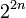# Quiz:Degrees of irreducible representations

3 What is the largest possible value of the maximum degree of irreducible representation for a group of order$2^{2n + 1}$ over a splitting field of characteristic zero (such as the field of complex numbers) where$n$ is a positive integer?
 2$2^n$$2^{n + 1}$$2^{2n - 1}$$2^{2n}$$2^{2n + 1}$